# Jacobian

(diff) ← Older revision | Latest revision (diff) | Newer revision → (diff)

Jacobi determinant

A determinant of a matrix of special form whose entries are first-order partial derivatives of functions. Let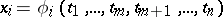,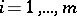, be given functions having first-order partial derivatives with respect to the variables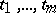. The Jacobian of these functions is the determinant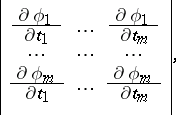which for brevity is denoted by the symbol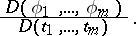The modulus of a Jacobian characterizes the dilatation (contraction) of the volume element in the transition from the variables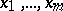to. The name is given after C.G.J. Jacobi, who first studied its properties and applications.

How to Cite This Entry:
Jacobian. Encyclopedia of Mathematics. URL: http://encyclopediaofmath.org/index.php?title=Jacobian&oldid=14954
This article was adapted from an original article by V.A. Il'in (originator), which appeared in Encyclopedia of Mathematics - ISBN 1402006098. See original article